Courses

# Automata with Output Computer Science Engineering (CSE) Notes | EduRev

## Computer Science Engineering (CSE) : Automata with Output Computer Science Engineering (CSE) Notes | EduRev

The document Automata with Output Computer Science Engineering (CSE) Notes | EduRev is a part of the Computer Science Engineering (CSE) Course Theory of Computation.
All you need of Computer Science Engineering (CSE) at this link: Computer Science Engineering (CSE)

Part VI. Automata with Output
Consider the following finite automation,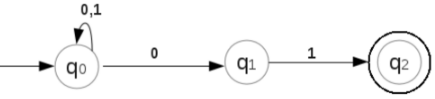Check whether the string 01101 is accepted by the above automation.
δ(q0, 01101) = q0
δ(q0, 01101) =q0
δ(q0, 01101) = q0
δ(q0, 01101) = q1
δ(q1, 01101) = q2
qis a final state. So the string 01101 is accepted by the above NFA.
Here note the output we obtained from the automation. THe output is that the string 01101 is accepted by the automation.Thus in our previous discussion on finite automata, there are only two possible outputs, ie, accept or reject. Thus here the task done by the machine is simply recognize a language. But machines can do more than this. So here we learn finite automata with output capabilities. We will learn two models of finite automata with output capabilities. They are,
Moore Machine, and
Mealy Machine.

10 Moore Machine
Moore machine is a finite automation.
In this finite automation, output is associated with every state.
In Moore machine, every state has a fixed output.

Example,
The following is an example transition table for a Moore Machine.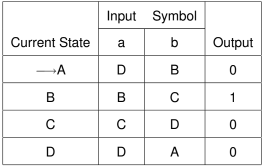The transition diagram corresponding to this is ,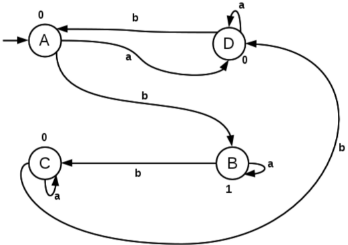A is the start state.
There is no final state in a Moore machine.
Output is shown above every state.

Definition of a Moore Machine
Moore Machine is a six tuple machine and is defined as,

M0 ( Q, ∑, ∆, δ, λ', q0 )
where M0 is the Moore Machine,
Q is a finite set of states,
∑ is a set of input symbols,
∆ is set of outputs,
δ is the set of transition functions,
λ'is the mapping function which maps a state to an output,
q0 is the start state.

Consider the following Moore Machine,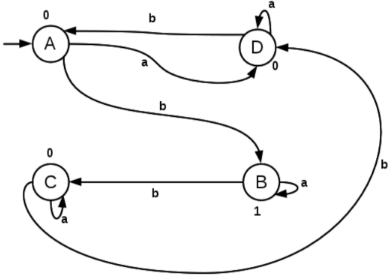In the above Moore Machine,

The set of states,
Q = {A, B, C, D}

The set of input symbols,
∑ = {a, b}

The set of outputs,
∆ = {0, 1}

The set of transitions,
δ(A, a) = D
δ(B, b) = C
δ(C, a) = C and so on.

λ'(A) = 0
λ'(B) = 1
λ'(C) = 0
λ'(D) = 0

String Processing through Moore Machine

Example 1:
Consider the Moore Machine given below: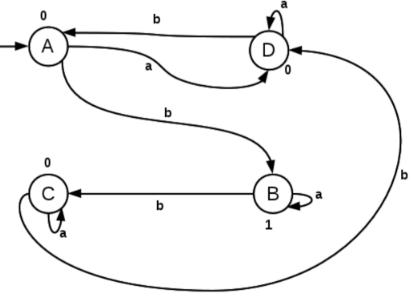Process the string abbb using the Moore Machine and find the output string.
Begin from the start symbol, A,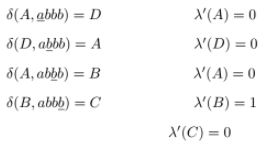The output string we got is 00010.

Example 2:
Consider the following Moore Machine,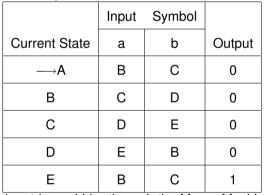Process the string aabbba through the Moore Machine and find the output.

Note that here transition table corresponding to the Moore Machine is given.
Begin from the start symbol, A,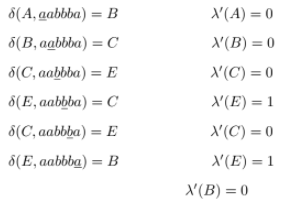The output string we got is 0001010.

11 Mealy Machine
Mealy machine is a finite automation.
In this finite automation, output is associated with every transition.
In Mealy machine, every transition for a particular input symbol has a fixed output.

Example,
The following is an example transition table for a Mealy Machine.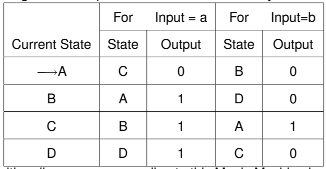The transition diagram corresponding to this Mealy Machine is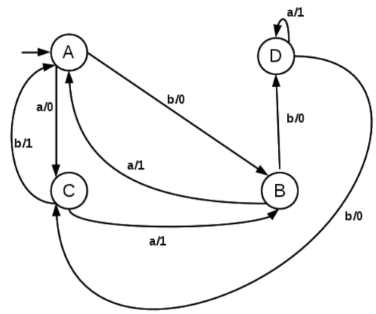A is the start state.
There is no final state in a Mealy machine.
Output is shown with every transition. (a/1 means a is the input symbol and output is 1).

Definition of a Mealy Machine
Mealy Machine is a six tuple machine and is defined as,

Me ( Q, ∑, ∆, δ, λ', q0 )
where Me is the Mealy Machine,
Q is a finite set of states,
∑ is a set of input symbols,
∆ is set of outputs,
δ is the set of transition functions,
λ'is the mapping function which maps a state and an input symbol to an output,
q0 is the start state.

Consider the following Mealy Machine,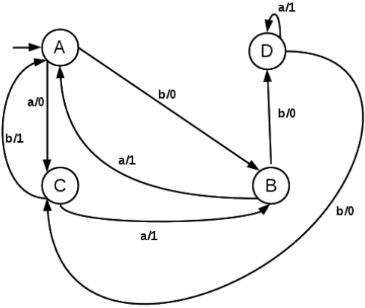In the above Moore Machine,

The set of states,
Q = {A, B, C, D}

The set of input symbols,
P = {a, b}

The set of outputs,
∆ = {0, 1}

The set of transitions,
δ(A, a) = C
δ(B, b) = D
δ(C, a) = B and so on.

λ'(A, a) = 0
λ'(B, a) = 1
λ'(C, a) = 1
λ'(D, b) = 0 and so on.

String Processing through Mealy Machine

Example 1:
Consider the Mealy Machine given below:Process the string abbb through the above Mealy Machine and find out the output.
Begin from the start symbol, A,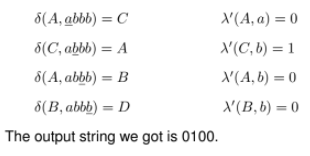12 Moore Machine to Mealy Machine Conversion

Example 1:
Consider the Moore Machine given below: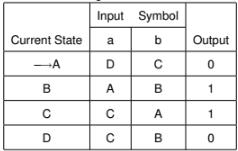Convert this Moore Machine to a Mealy Machine.

If we look at the above transition table, we can see that the machine has output 0 for the states A and D. The machine has output 1 for the states B and C.

To convert this to a Mealy Machine, the output is taken as 0, whenever there is a transition to A or D. Also, the output is taken as 1, whenver there is a transition to B or C.

The Mealy Machine is as follows: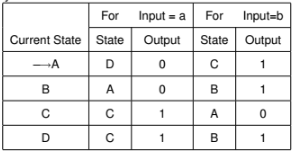Example 2:
Convert the Moore Machine given below to a Mealy Machine: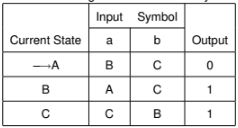If we look at the above transition table, we can see that the machine has output 0 for the state A. The machine has output 1 for the states B and C.

To convert this to a Mealy Machine, the output is taken as 0, whenever there is a transition to A. Also, the output is taken as 1, whenver there is a transition to B or C.

The Mealy Machine is as follows: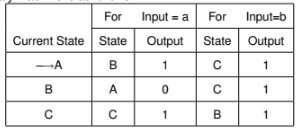13 Mealy Machine to Moore Machine Conversion

Example 1:
Consider the Mealy Machine given below: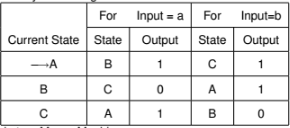Conver this to a Moore Machine.

Here, State A has output 1 at two places.
State B has output 0 at one place and 1 at another place. So this state B is decomposed into two states, B0 and B1. In the new Moore Machine, state B0 has output 0 and B1 has output 1.
State C has output 0 at one place and output 1 at another place. So this state is decomposed into two states, C0 and C1. In the new Moore Machine, state C0 has output 0 and C1 has output 1.

The Moore Machine is given below;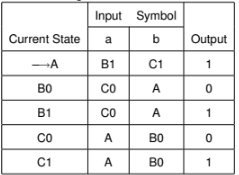Example 2:
Convert the following Mealy Machine to Moore Machine.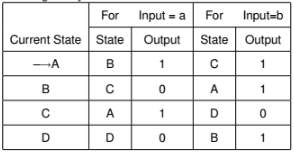Here, State A has output 1 at two places.
State B has output 1 at two places.
State C has output 0 at one place and output 1 at another place. So this state is decomposed into two states, C0 and C1. In the new Moore Machine, state C0 has output 0 and C1 has output 1.
State D has output 0 at both places.

The Moore Machine is given below;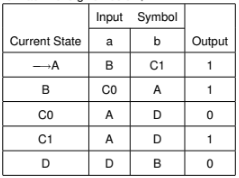Offer running on EduRev: Apply code STAYHOME200 to get INR 200 off on our premium plan EduRev Infinity!

## Theory of Computation

18 videos|43 docs|39 tests

,

,

,

,

,

,

,

,

,

,

,

,

,

,

,

,

,

,

,

,

,

;